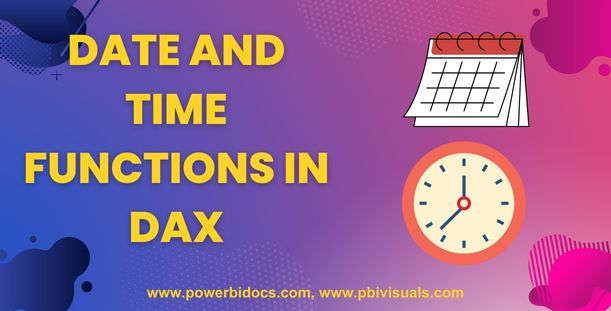Home » DAX » Exploring DateTime Functions in DAX: Syntax, Examples, and Common Queries

# Exploring DateTime Functions in DAX: Syntax, Examples, and Common Queries### 1- TODAY DAX Function

Today() returns the current date.

Syntax: TODAY()

Example: Calculates the sum of sales amount for the current day.

```TodaySales = CALCULATE(SUM(SalesTable[SalesAmount]),
SalesTable[Date] = TODAY())```

### 2- NOW() DAX Function

The NOW function in DAX is used to obtain the current date and time as a datetime value.

Syntax: NOW()

Example:

`CurrentDateTime = NOW()`

In this example, `NOW()` is called without any arguments. The function retrieves the current date and time when the calculation is executed.

### 3- YEAR DAX Function

YEAR(date) returns the year from the specified date.

Syntax: YEAR()

Example: Calculates the sum of sales amount for the year 2023.

```SalesByYear = CALCULATE(SUM(SalesTable[SalesAmount]),
YEAR(SalesTable[Date]) = 2023)```

### 4- MONTH DAX Function

Month(date) returns the month from the specified date.

Syntax: MONTH()

Example: Calculates the sum of sales amount for the month of June.

```SalesByMonth = CALCULATE(SUM(SalesTable[SalesAmount]),
MONTH(SalesTable[Date]) = 6)```

### 5- DAY DAX Function

DAY(date) returns the day from the specified date.

Syntax: DAY()

Example: Calculates the sum of sales amount for the 17th day of the month.

```SalesByDay = CALCULATE(SUM(SalesTable[SalesAmount]),
DAY(SalesTable[Date]) = 17)```

### 6- EOMONTH DAX function

EOMONTH() returns the end of the month for the specified start_date.

It takes two arguments- the start_date and months. The start_date is the date for which we want to find the end of the month, and the months argument is optional and specifies the number of months to add or subtract from the start_date.

Syntax: EOMONTH(start_date, months)

Example: How to get the last day of the month for the specified date?

`LastDayOfMonth = EOMONTH(DATE(2023, 6, 17), 0)`

In this case, the start_date is `DATE(2023, 6, 17)`, representing June 17, 2023. The months argument is set to 0, which means no additional months are added or subtracted.

In this example, the result will be the date `June 30, 2023`, as it is the last day of the month for `June 17, 2023`.

### 7- EDATE DAX Function

The EDATE function in DAX (Data Analysis Expressions) is used to calculate a new date by adding or subtracting a specified number of months from a given date.

Syntax: EDATE(start_date, months)

• start_date: The initial date from which the calculation begins.
• months: The number of months to add or subtract from the start_date. Positive values represent future dates, and negative values represent past dates.

Example 1:

Create a Measure with below code-

`NewDate = EDATE(DATE(2023, 6, 5),  1)`

So, the expression `EDATE(DATE(2023, 6, 5), 1)` returns the date `July 5, 2023.`

Example 2:

Add New column and write below dax code-

`New-Date = EDATE('Sales'[OrderDate], 1)`

In this example, `'Sales'[OrderDate]` represents the column that contains the initial dates.

The `EDATE` function takes the `"OrderDate"` and adds `1` months to it. The resulting date will be stored in the `"New-Date"` column.

### 8- CALENDAR DAX Function

The CALENDAR function is used to create a calendar table that includes a continuous range of dates.

This function is commonly used to generate a table with a list of dates to support time-based calculations and analysis.

Syntax: CALENDAR(start_date, end_date)

Example: Generate a calendar table named “CalendarTable” that includes all the dates from January 1, 2023, to December 31, 2023.

```CalendarTable = CALENDAR( DATE(2023, 1, 1),
DATE(2023, 12, 31)
)```

You can further enhance the calendar table by adding additional columns such as year, month, day of the week, or other derived date-related information using DAX functions like YEAR(), MONTH() etc.

```CalendarTable =
CALENDAR( DATE(2023, 1, 1),  DATE(2023, 12, 31)),
"Year", YEAR([Date]),
"Month", MONTH([Date])
)```

### 9- CALENDARAUTO DAX Function

Returns a table with a single column named “Date” that contains a contiguous set of dates. The range of dates is calculated automatically based on data in the model.

Syntax: CALENDARAUTO()

Example:

`CalendarTable = CALENDARAUTO()`

CALENDARAUTO() will scan the entire data model and identify a column with a date or datetime data type.

It will determine the minimum and maximum dates from that column and generate a calendar table covering the range of dates.

### 10- DATEDIFF DAX Function

The DATEDIFF function is used to calculate the difference between two dates in terms of a specified time unit.

It returns the number of time units (such as days, months, or years) between the start and end dates.

Syntax: DATEDIFF(start_date, end_date, time_unit)

Example:

```DaysDifference = DATEDIFF(DATE(2023, 1, 1),
DATE(2023, 12, 31), DAY)```

In this example, the DATEDIFF function calculates the number of days between January 1, 2023 (start_date) and December 31, 2023 (end_date).

The time_unit parameter is set to `"DAY"`, indicating that we want to measure the difference in terms of days.

Additionally, you can use other time_unit options such as `"MONTH"`, `"YEAR"`, `"QUARTER"` etc.

### 11- WEEKDAY DAX Function

The WEEKDAY function in DAX is used to determine the day of the week for a given date and return its corresponding numeric representation or name.

Syntax: WEEKDAY(date, [return_type])

• date: The date for which you want to determine the day of the week.
• Return type: 1, week begins on Sunday (1) and ends on Saturday (7). numbered 1 through 7.
Return type: 2, week begins on Monday (1) and ends on Sunday (7).
Return type: 3, week begins on Monday (0) and ends on Sunday (6).numbered 1 through 7.

Example:

`DayOfWeek = WEEKDAY('Sales'[OrderDate], 1)`

### 12- HOUR DAX Function

The HOUR function in DAX is used to extract the hour component from a datetime value.

Syntax: HOUR(datetime)

Example 1:

Create a New Column to dataset and write below dax-

`OrderHour = HOUR('Sales'[OrderDateTime])`

In this example, `'Sales'[OrderDateTime]` represents the column that contains the datetime values. The `HOUR` function is applied to each datetime value, extracting the hour component.

Example 2:

Create a Measure and write below DAX code-

`Hour("2023-06-17 15:30:00")`
It will extract the hour component, and returns the value 15.
Hope you enjoyed the post, you can refer Other DAX functions: DAX functions